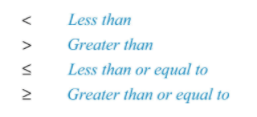## OBJECTIVE: To learn how to graph inequalities on the real number line

In previous lessons, you learned about the set of real numbers and their subsets. You also learned how to construct the real number line, graph numbers on it, and compare numbers based on their positions in the number line. Keeping It Real: https://www.geogebra.org/m/n8hauvp6 Keeping in Line: https://www.geogebra.org/m/sw4yxv53 Calling to Order: https://www.geogebra.org/m/vrfcsfut In this lesson, you'll learn how to graph inequalities as subsets of real numbers on the real number line.

## HOW TO GRAPH INEQUALITIES AS SUBSETS OF REAL NUMBERS ON THE REAL NUMBER LINE

Here are the common symbols used to show order relationships between real numbers. Familiarize yourself with them.Below are some examples of graphs of subsets of the set of real numbers on the real number line.
Use the applet below to see dynamic illustrations of graphing subsets of real numbers on the real number line. Subsets are given in both interval notation and inequality notation. Move the slider to see various possibilities for the inequalities. Drag the two dots to change the boundaries.

## TRY IT YOURSELF ...

Practice graphing subsets of real numbers using the applet below. This applet includes one-sided inequalities, conjunctions, and disjunctions. Repeat the exercise as often as needed to master the concept.